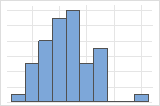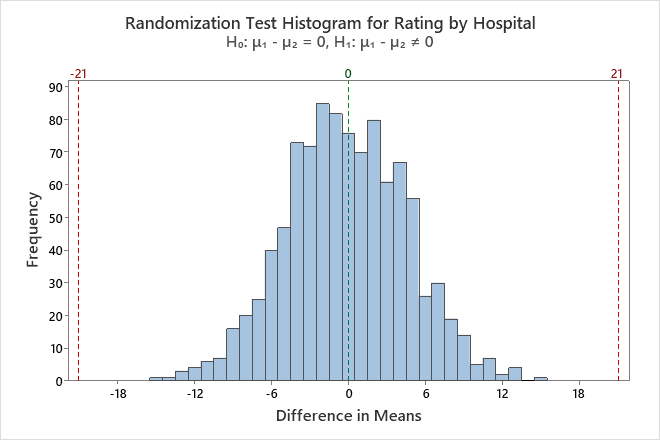# Randomization test statistics and graphs for Randomization test for 2-sample means

Find definitions and interpretation guidance for every randomization test statistic and graph that is provided with randomization test for 2-sample mean.

## Histogram

A histogram divides sample values into many intervals and represents the frequency of data values in each interval with a bar.

### Interpretation

Use the histogram to examine the shape of your bootstrap distribution. The bootstrap distribution is the distribution of the chosen statistic from each resample. The bootstrap distribution should appear to be normal. If the bootstrap distribution is non-normal, you cannot trust the bootstrap results.The distribution is usually easier to determine with more resamples. For example, in these data, the distribution is ambiguous for 50 resamples. With 1000 resamples, the shape looks approximately normal.

The histogram visually shows the results of the hypothesis test. The randomization samples represent what a random sample would look like if the population means were equal, so the histogram is centered around 0. For a one-sided test, a reference line is drawn at the difference in means of the original sample. For a two-sided test, a reference line is drawn at the difference in means of the original sample and at the same distance on the opposite side of 0. The p-value is the proportion of sample differences that are more extreme than the values at the reference lines. In other words, the p-value is the proportion of sample differences that are as extreme as your original sample when you assume that the null hypothesis is true. These differences are colored red on the histogram.In this histogram, the bootstrap distribution appears to be normal. None of the sample differences are greater than 21 or less than -21.

## Individual value plot

An individual value plot displays the individual values in the sample. Each circle represents one observation. An individual value plot is especially useful when you have relatively few observations and when you also need to assess the effect of each observation.

###### Note

Minitab displays an individual value plot only when you take only one resample. Minitab displays both the original data and the resample data.

### Interpretation

Use the individual value plot to compare the original samples and the randomization samples. The randomization samples represent what a random sample would look like if the population means were equal. Thus, the line that connects the means of the randomization samples tends to be flat. Compare the difference in means of the original samples to the difference in the means of the randomization samples. The steeper the line between the observed samples relative to the line between the randomization samples, the more evidence you would expect against the null hypothesis.

## Null hypothesis and alternative hypothesis

The null and alternative hypotheses are two mutually exclusive statements about a population. A hypothesis test uses sample data to determine whether to reject the null hypothesis.
Null hypothesis
The null hypothesis states that a population parameter (such as the mean, the standard deviation, and so on) is equal to a hypothesized value. The null hypothesis is often an initial claim that is based on previous analyses or specialized knowledge.
Alternative hypothesis
The alternative hypothesis states that a population parameter is smaller, greater, or different than the hypothesized value in the null hypothesis. The alternative hypothesis is what you might believe to be true or hope to prove true.

## Number of Resamples

The number of resamples is the number of times Minitab takes a random sample with replacement from your original data set. Usually, a large number of resamples works best. The sample size for each resample is equal to the sample size of the original data set. The number of resamples equals the number of observations on the histogram.

## Average

The average is the sum of all the differences in means of the randomization sample divided by the number of resamples. Minitab displays two different values for the difference in means, the difference of the observed samples and the difference of the bootstrap distribution (Average). Both these values are an estimate of the difference in population means and will usually be similar. If there is a large difference between these two values, you should increase the sample size of your original sample.

## StDev (bootstrap sample)

The standard deviation is the most common measure of dispersion, or how spread out the data are about the mean. The symbol σ (sigma) is often used to represent the standard deviation of a population, while s is used to represent the standard deviation of a sample. Variation that is random or natural to a process is often referred to as noise. Because the standard deviation is in the same units as the data, it is usually easier to interpret than the variance.

The standard deviation of the bootstrap samples (also known as the bootstrap standard error) is an estimate of the standard deviation of the sampling distribution of the difference in means.

### Interpretation

Use the standard deviation to determine how spread out the differences from the bootstrap sample are from the overall mean of the differences. A higher standard deviation value indicates greater spread in the differences. A good rule of thumb for a normal distribution is that approximately 68% of the values fall within one standard deviation of the overall mean of the differences, 95% of the values fall within two standard deviations, and 99.7% of the values fall within three standard deviations.

Use the standard deviation of the bootstrap samples to determine how precisely the differences from the bootstrap sample estimate the population difference in means. A smaller value indicates a more precise estimate of the population difference. Usually, a larger standard deviation results in a larger bootstrap standard error and a less precise estimate of the population difference. A larger sample size results in a smaller bootstrap standard error and a more precise estimate of the population difference.

## P-Value

The p-value is the proportion of sample differences that are as extreme as your original sample when you assume that the null hypothesis is true. A smaller p-value provides stronger evidence against the null hypothesis.

### Interpretation

Use the p-value to determine whether the difference in population means is statistically significant. To determine whether the difference between the population means is statistically significant, compare the p-value to the significance level. Usually, a significance level (denoted as α or alpha) of 0.05 works well. A significance level of 0.05 indicates a 5% risk of concluding that a difference exists when there is no actual difference.
P-value ≤ α: The difference between the means is statistically significant (Reject H0)
If the p-value is less than or equal to the significance level, the decision is to reject the null hypothesis. You can conclude that the difference between the population means is statistically significant. To calculate a confidence interval and determine whether the difference is practically significant, use Bootstrapping for 2-sample means. For more information, go to Statistical and practical significance.
P-value > α: The difference between the means is not statistically significant (Fail to reject H0)
If the p-value is greater than the significance level, the decision is to fail to reject the null hypothesis. You do not have enough evidence to conclude that the difference between the population means is statistically significant.
By using this site you agree to the use of cookies for analytics and personalized content.  Read our policy